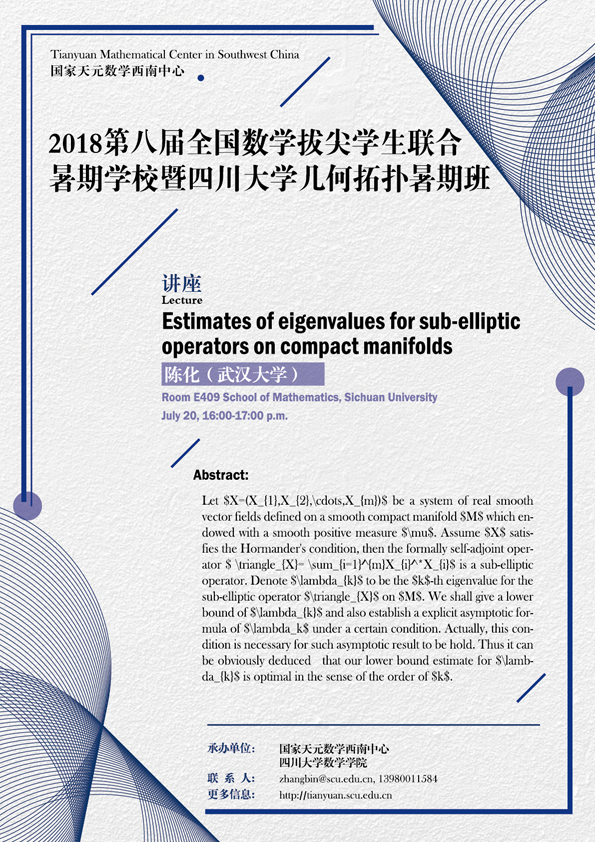### Estimates of eigenvalues for sub-elliptic operators on compact manifolds

#### E409  School of Mathematics#### ABSTRACT

Let $X=(X_{1},X_{2},\cdots,X_{m})$ be a system of real smooth vector fields defined on a smooth compact manifold $M$ which endowed with a smooth positive measure $\mu$. Assume $X$ satisfies the Hormander's condition, then the formally self-adjoint operator $\triangle_{X}=-\sum_{i=1}^{m}X_{i}^*X_{i}$ is a sub-elliptic operator. Denote $\lambda_{k}$ to be the $k$-th eigenvalue for the sub-elliptic operator $\triangle_{X}$ on $M$. We shall give a lower bound of $\lambda_{k}$ and also establish a explicit asymptotic formula of $\lambda_k$ under a certain condition. Actually, this condition is necessary for such asymptotic result to be hold. Thus it can be obviously deduced that our lower bound estimate for $\lambda_{k}$ is optimal in the sense of the order of $k$.

#### SUPPORTED BY

Tianyuan Mathematical Center in Southwest China

School of Mathematics, Sichuan University